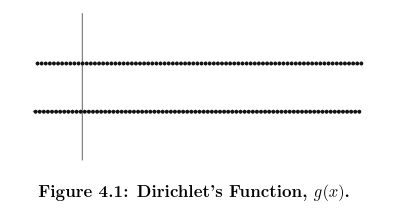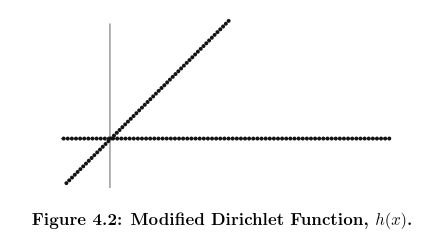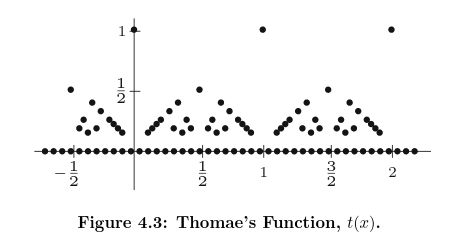Show Question
Math and science::Analysis::Tao::09. Continuous functions on R

# Continous functions

Let $$X$$ be a subset of $$\mathbb{R}$$, and let $$f: X \to \mathbb{R}$$ be a function. Let $$x_0$$ be an element of $$X$$. We say that $$f$$ is continuous at $$x_0$$ iff we have:

$\lim_{x \to x_0; x \in X} f(x) = f(x_0);$

in other words, the limit of $$f(x)$$ as $$x$$ converges to $$x_0$$ in X exists and is equal to $$f(x_0)$$.

We say that $$f$$ is continuous on $$X$$ (or simply continuous) iff $$f$$ is continuous at $$x_0$$ for every $$x_0 \in X$$. We say that $$f$$ is discontinous at $$x_0$$ iff it is not continous at $$x_0$$.

Tao describes this definition as one of the most fundamental notions in the theory of functions.

Question: what is Dirichlet's function and what is Thomae's function? How do they relate to function continuity?

### Essence of continuity

A collection of ideas that suggest ways of thinking about function limits and continuity.

Maybe some or all of these would be better on the card for function limits.

#### Nearness, maintained through function application

Abbott:
Given a function $$f$$ with domain $$A \subset \mathbb{R}$$, we want to define continuity at a point $$c \in A$$ to mean that if $$x \in A$$ is chosen near $$c$$, then $$f(x)$$ will be near $$f(c)$$.

#### Dirichlet's functionTo know what something is, it can be best to investigate what it isn't. This line of thinking brings us to some cases that test the notion of continuity.

Peter Lejeune Dirichlet defined the function $$g$$ based on whether the input variable is rational or irrational:

$g(x) = \begin{cases} 1 &\quad \text{if } x \in \mathbb{Q} \\ 0 &\quad \text{if } x \notin \mathbb{Q} \\ \end{cases}$

Of this function, does it make sense to give meaning to the following limit: $$\lim_{x \to \frac{1}{2}} g(x)$$? One idea is to consider this to represent the limit of the sequence $$(g(x_n))_{n=0}^{\infty}$$. But for this expression, we must decide the sequence $$(x_n)_{n=0}^{\infty}$$, and it can be seen that this choice leads different possible sequences $$(g(x_n))_{n=0}^{\infty}$$. For example, if each $$x_n$$ is rational, then:

$\lim_{n\to\infty} g(x_n) = 1$

whereas, if each $$x_n$$ is irrational, then:

$\lim_{n\to\infty} g(x_n) = 0$

Our naive definition of function limits is clearly insufficient; if we were to assign a value to the expression $$\lim_{n\to c} g(x)$$ in terms of sequences in the domain, we would want the value to be independent of how we approach $$c$$. Whatever the definition of the function limit, it seems fair to say that the example above, $$\lim_{x \to \frac{1}{2}} g(x)$$, should not be given a value.

Not only at $$\frac{1}{2}$$, but at all points of the domain of Dirichlet's we see that the function fails to meet our intuitive idea of continuity. Realize that both $$\mathbb{Q}$$ and $$\mathbb{I}$$ are both dense in $$\mathbb{R}$$, and so any point in $$\mathbb{R}$$ can be expressed as a limit of a sequence confined to $$\mathbb{Q}$$ or a sequence confined to $$\mathbb{I}$$.

Dirichlet's function is said to be a nowhere-continuous function on $$\mathbb{R}$$.

#### Sequence independenceWhat happens if we change the definition of $$g$$ like so:

$g(x) = \begin{cases} x &\quad \text{if } x \in \mathbb{Q} \\ 0 &\quad \text{if } x \notin \mathbb{Q} \\ \end{cases}$

If $$c$$ is not zero, then we are in the same situation as before; but if $$c = 0$$, then the sequence $$g(x_n)_{n=0}^{\infty}$$ approaches zero in both the case where $$\forall n \in \mathbb{N}, x_n \in \mathbb{Q}$$ and where $$\forall n \in \mathbb{N}, x_n \in \mathbb{I}$$.

We should be more comfortable considering assigning the value 0 to the expression $$\lim_{x \to 0}g(x)$$. This observation gets at the heart of what it should mean for a function limit to exist. We really want

$\lim_{x \to c} f(x) = L \quad \text{iff} \quad f(x_n) \to L \; \text{ for any sequences } (x_n) \text{ such that } \lim_{n\to \infty}{x_n} = c$

#### Thomae's functionThe eponymously named sequence:

$t(x) = \begin{cases} 1 &\quad \text{if } x = 0 \\ \frac{1}{n} &\quad \text{if } x = \frac{m}{n} \in \mathbb{Q} \setminus \{0\} \text{ is in lowest terms, with } n > 0 \\ 0 &\quad \text{if } x \notin \mathbb{Q} \\ \end{cases}$

The last term means that any sequence $$(t(x_n))$$ for irrational sequences $$(x_n)$$ will always approach (be) $$0$$. This includes sequences of irrationals that approach a rational. Thus, the last term insures that $$t$$ is not continuous at rational points.

In comparison, the effect of the middle term is to send $$t(x)$$ towards zero for sequences of rationals $$(x)$$ that approach an irrational. But $$t$$ at an irrational point is 0 anyway, so we find that $$t$$ seems to be continuous at irrational points.

Thus, Thomae's function has the strange property of being continuous at all irrational points, yet discontinuous at all rational points.

### Historical perspective

Abbott mentions in Understanding Analysis that the concept of continuity came long after (~200 years) the derivative was in wide use; the interesting point being that this is now in contrast to the order in which the subjects are taught.

Abbott explains that the concept of a function was, for a long time, more restricted and did not permit discontinuities. When Fourier series (limits of sums of continuous functions) began to be used to represent discontinuous functions, the question arose: does the continuity of the polynomials being summed not imply the continuity of the limit of the sum? Such questions required the idea of continuity to have a definition that was more precise than "no holes".

### Constant function

Let $$f : \mathbb{R} \to \mathbb{R}$$ be the constant function $$f(x) := c$$, for some constant $$c$$. Then for every real $$x_0 \in \mathbb{R}$$, we have

$\lim_{x\to x_0; x\in \mathbb{R}}f(x) = \lim_{x \to x_0; x \in \mathbb{R}}c = c = f(x_0)$

Thus, $$f$$ is continuous at every point $$x_0 \in \mathbb{R}$$, or in other words $$f$$ is continuous on $$\mathbb{R}$$.

### Identity function

Let $$f : \mathbb{R} \to \mathbb{R}$$ be the identity function $$f(x) := x$$, for some constant $$c$$. Then for every real $$x_0 \in \mathbb{R}$$, we have

$\lim_{x\to x_0; x\in \mathbb{R}}f(x) = \lim_{x \to x_0; x \in \mathbb{R}}x = x_0 = f(x_0)$

Thus, $$f$$ is continuous at every point $$x_0 \in \mathbb{R}$$, or in other words $$f$$ is continuous on $$\mathbb{R}$$.

### Signum function

Let $$f : \mathbb{R} \to \mathbb{R}$$ be the signum function:

$sgn(x) := \begin{cases} 1, &\quad \text{if } x > 0 \\ 0, &\quad \text{if } x = 0 \\ -1 &\quad \text{if } x < 0 \\ \end{cases}$

Then $$sgn(x)$$ is continuous at every non-zero value of $$x$$, for instance , at 1:

\begin{aligned} \\ \lim_{x \to 1; x \in \mathbb{R}} sgn(x) &= \lim_{x \to 1; x \in (0.9, 1.1)} sgn(x) \\ &= \lim_{x \to 1; x \in (0.9, 1.1)} 1 \quad \text{(as limits are local)} \\ &= 1 \\ &= sgn(1) \\ \end{aligned}

On the other hand, $$sgn$$ is not continuous at 0, since the limit there doesn't exist.

Tao has some other nice examples.

Tao, p228
Abbott, p112